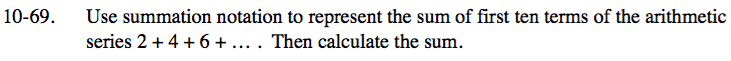### Home > INT3 > Chapter Ch10 > Lesson 10.1.5 > Problem10-69

10-69.

Use summation notation to represent the sum of first ten terms of the arithmetic series 2 + 4 + 6 + … . Then calculate the sum. Homework Help ✎$\sum_{n=1}^{10}(2n)$